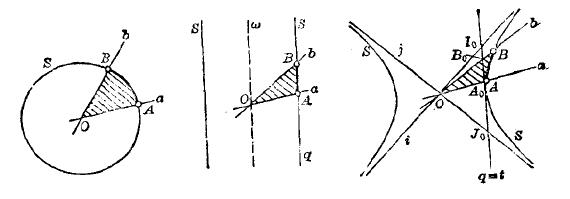# -*-紫色王家思絮絮-*-## 闲侃 (5)：三种时空变换对应的几何结构

(2013-11-19 08:45:11) 下一个

a) 速度可以是任意值；时间是绝对的，时空的变换是抛物型的；
b) 速度存在个上限值；时空的变换是双曲型的；
c) 速度可以是任意值；时空的变换是椭圆型的。

 角度测量分类 长度测量分类 椭圆型 抛物型 双曲型 椭圆型 椭圆几何学 欧几里得几何学 双曲几何学 抛物型 伴欧几里得几何学 加利略几何学 伴闵可夫斯基几何学 双曲型 伴双曲几何学 闵可夫斯基几何学 二重双曲几何学

Case c) 椭圆型络仑兹变换。考虑二维时空，我们有如下的变换：
t1 = b * (    t + v*x)

x1 = b * (-v*t +   x)

(t1, x1)' = A (t, x)'，A_11 = b，A_12 = bv，A_21 = -bv，A_22 = b，这里 b = 1/sqrt (1+v^2)，v 是速度，可以取任意值，包括 0。如前所说，椭圆型络仑兹

A_11 = cos w，     A_12 = sin w，
A_21 = -sin w，     A_22 = cos w。

A(w1)*A(w2) = A(w1+w2)(欧几里得几何、加利略几何、闵可夫斯基几何的单位圆和角度示意图
角度就是阴影部分的面积)

Case a) 迦利略变换，我们有如下的变换：
t1 =      t
x1 = x - vt

(t1, x1)' = A (t, x)'，
A_11 =1，      A_12 = 0，
A_21 = -v，    A_22 = 1，
v 是速度，可以是任意值，包括 0 和无穷大。如前所说，迦利略变换保持时间 t 不变，和 x 完全无关。很容易验证：
A(v1)*A(v2) = A(v1+v2)

Case b) 络仑兹变换。(rescale 使得最大速度 -- 光速 = 1)：
t1 = a*(      t  -  v*x)
x1 = a*(-v*t  +    x)

(t1, x1)' = A (t, x)'，
A_11 = a，       A_12 = -av，
A_21 = -av，     A_22 = a

sinh x = (e^x - e^(-x)) / 2; cosh x = (e^x + e^(-x)) / 2; tanh x = sinh x/cosh x；

A_11 = cosh w，        A_12 = -sinh w，
A_21 = -sinh w，        A_22 = cosh w

[ 打印 ]